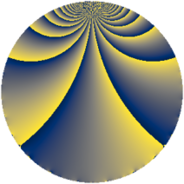# Properties

 Label 192.2.kLevel $192$ Weight $2$ Character orbit 192.k Rep. character $\chi_{192}(47,\cdot)$ Character field $\Q(\zeta_{4})$ Dimension $12$ Newform subspaces $1$ Sturm bound $64$ Trace bound $0$

# Related objects

## Defining parameters

 Level: $$N$$ $$=$$ $$192 = 2^{6} \cdot 3$$ Weight: $$k$$ $$=$$ $$2$$ Character orbit: $$[\chi]$$ $$=$$ 192.k (of order $$4$$ and degree $$2$$) Character conductor: $$\operatorname{cond}(\chi)$$ $$=$$ $$48$$ Character field: $$\Q(i)$$ Newform subspaces: $$1$$ Sturm bound: $$64$$ Trace bound: $$0$$

## Dimensions

The following table gives the dimensions of various subspaces of $$M_{2}(192, [\chi])$$.

Total New Old
Modular forms 80 20 60
Cusp forms 48 12 36
Eisenstein series 32 8 24

## Trace form

 $$12q + 2q^{3} + 8q^{7} + O(q^{10})$$ $$12q + 2q^{3} + 8q^{7} - 4q^{13} + 12q^{19} - 8q^{21} - 10q^{27} - 4q^{33} - 4q^{37} - 20q^{39} - 12q^{43} - 12q^{45} - 20q^{49} - 24q^{51} - 24q^{55} + 12q^{61} - 28q^{67} + 4q^{69} + 34q^{75} - 4q^{81} + 32q^{85} + 60q^{87} + 56q^{91} + 28q^{93} - 8q^{97} + 52q^{99} + O(q^{100})$$

## Decomposition of $$S_{2}^{\mathrm{new}}(192, [\chi])$$ into newform subspaces

Label Dim. $$A$$ Field CM Traces $q$-expansion
$$a_2$$ $$a_3$$ $$a_5$$ $$a_7$$
192.2.k.a $$12$$ $$1.533$$ 12.0.$$\cdots$$.2 None $$0$$ $$2$$ $$0$$ $$8$$ $$q-\beta _{10}q^{3}+\beta _{7}q^{5}+(1-\beta _{11})q^{7}+(\beta _{3}+\cdots)q^{9}+\cdots$$

## Decomposition of $$S_{2}^{\mathrm{old}}(192, [\chi])$$ into lower level spaces

$$S_{2}^{\mathrm{old}}(192, [\chi]) \cong$$ $$S_{2}^{\mathrm{new}}(48, [\chi])$$$$^{\oplus 3}$$